• 在监督式机器学习中，无论是回归问题还是分类问题，都少不了使用损失函数（Loss Function）。**损失函数（Loss Function）**是用来估量模型的预测值 f(x) 与真实值 y 的不一致程度。若损失函数很小，表明机器学习...
原文地址：https://redstonewill.com/1584/  （转自红色石头）
前言
在监督式机器学习中，无论是回归问题还是分类问题，都少不了使用损失函数（Loss Function）。**损失函数（Loss Function）**是用来估量模型的预测值 f(x) 与真实值 y 的不一致程度。若损失函数很小，表明机器学习模型与数据真实分布很接近，则模型性能良好；若损失函数很大，表明机器学习模型与数据真实分布差别较大，则模型性能不佳。我们训练模型的主要任务就是使用优化方法来寻找损失函数最小化对应的模型参数。
在讨论分类问题的损失函数之前，我想先说一下模型的输出 g(s)。一般来说，二分类机器学习模型包含两个部分：线性输出 s 和非线性输出 g(s)。其中，线性输出一般是模型输入 x 与 参数 w 的乘积，简写成：s = wx；非线性输出一般是 Sigmoid 函数，经过 Sigmoid 函数，g(s) 值被限定在 [0,1] 之间，若 s ≥ 0，g(s) ≥ 0.5，则预测为正类；若 s < 0，g(s) < 0.5，则预测为负类。
关于正类和负类的表示，通常有两种方式：一种是用 {+1, -1} 表示正负类；另一种是用 {1, 0} 表示正负类。下文默认使用 {+1, -1}，因为这种表示方法有种好处。如果使用 {+1, -1} 表示正负类，我们来看预测类别与真实类别的四种情况：

s ≥ 0, y = +1: 预测正确
s ≥ 0, y = -1: 预测错误
s < 0, y = +1: 预测错误
s < 0, y = -1: 预测正确

显然，上面四个式子可以整合成直接看 ys 的符号即可：

若 ys ≥ 0，则预测正确
若 ys < 0，则预测错误

这里的 ys 类似于回归模型中的残差y-s(其中yg(s)被称为函数间隔（“margin”）)；最小化分类问题中的损失函数可以看做是最大化margin的过程，任何合格的分类损失函数都应该对margin<0的样本更大的“惩罚”。因此，在比较分类问题的各个损失函数的时候，我们就可以把 ys 当作自变量 x 轴即可，这样更加方便。
0-1 Loss
0-1 Loss 是最简单也是最容易直观理解的一种损失函数。对于二分类问题，如果预测类别 y_hat 与真实类别 y 不同（样本分类错误），则 L=1；如果预测类别 y_hat 与 真实类别 y 相同（样本分类正确），则 L=0（L 表示损失函数）。0-1 Loss 的表达式为：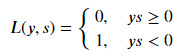0-1 Loss 的曲线如下图所示：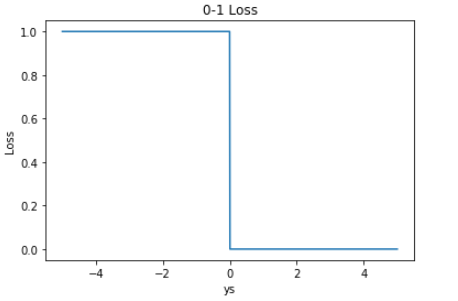0-1 Loss 的特点就是非常直观容易理解。但是它存在两个缺点：

0-1 Loss 对每个错分类点都施以相同的惩罚（损失为 1），这样对犯错比较大的点（ys 远小于 0，极端一点即margin接近负无穷）无法进行较大的惩罚，所有犯错点都同等看待，这不符合常理，不太合适。
0-1 Loss 不连续、非凸、不可导，难以使用梯度优化算法。
因此，实际应用中，0-1 Loss 很少使用。

Cross Entropy Loss
Cross Entropy Loss 是非常重要的损失函数，也是应用最多的损失函数之一。二分类问题的交叉熵 Loss 主要有两种形式（标签y的定义不同）：
（1）基于输出标签 label 的表示方式为 {0,1}，也最为常见。它的 Loss 表达式为：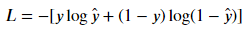推导过程：
从极大似然性的角度出发，预测类别的概率可以写成：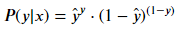当真实样本标签 y = 1 时，上面式子第二项就为 1，概率等式转化为：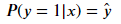当真实样本标签 y = 0 时，上面式子第一项就为 1，概率等式转化为：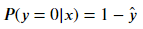我们希望的是概率 P(y|x) 越大越好。首先，我们对 P(y|x) 引入 log 函数，因为 log 运算并不会影响函数本身的单调性。则有：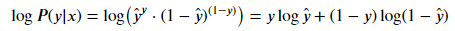希望 log P(y|x) 越大越好，反过来，只要 log P(y|x) 的负值 -log P(y|x) 越小就行了。那我们就可以引入损失函数，且令 Loss = -log P(y|x) 即可。则得到损失函数为：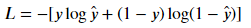我们来看，当 y = 1 时：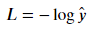代入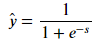得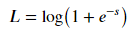这时候，Loss 的曲线如下图所示：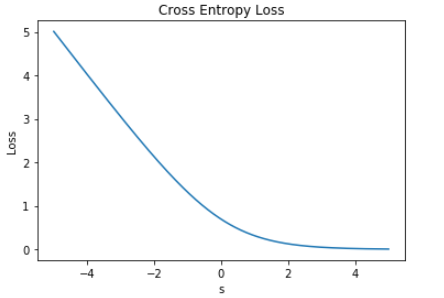从图中明显能够看出，s 越大于零，L 越小，函数的变化趋势也完全符合实际需要的情况；
当 y = 0 时：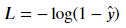对上式进行整理，同样能得到：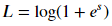这时候，Loss 的曲线如下图所示：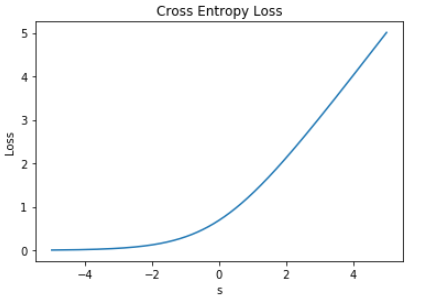从图中明显能够看出，s 越小于零，L 越小，函数的变化趋势也完全符合实际需要的情况。
（2）基于输出标签 label 的表示方式为 {-1,+1} （Logistics Loss），也比较常见。它的 Loss 表达式为：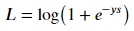下面对上式做个简单的推导，我们在 0 小节说过，ys 的符号反映了预测的准确性。除此之外，ys 的数值大小也反映了预测的置信程度。所以，从概率角度来看，预测类别的概率可以写成：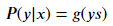接下来，同样引入 log 函数，要让概率最大，反过来，只要其负数最小即可。那么就可以定义相应的损失函数为：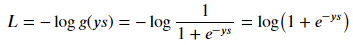这时候，我们以 ys 为横坐标，可以绘制 Loss 的曲线如下图所示：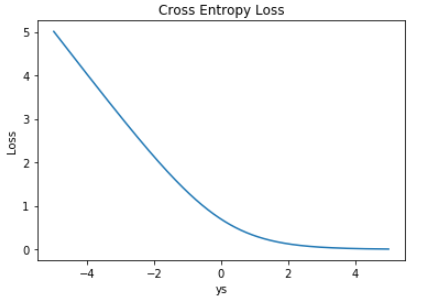其实上面介绍的两种形式的交叉熵 Loss 是一样的，只不过由于标签 label 的表示方式不同，公式稍有变化。标签用 {-1,+1} 表示的好处是可以把 ys 整合在一起，作为横坐标，容易作图且具有实际的物理意义。
总结一下，交叉熵 Loss 的优点是在整个实数域内，Loss 近似线性变化。尤其是当 ys << 0 的时候，Loss 更近似线性。这样，模型受异常点的干扰就较小。 而且，交叉熵 Loss 连续可导，便于求导计算，是使用最广泛的损失函数之一。
Hinge Loss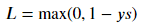Hinge Loss 的曲线如下图所示：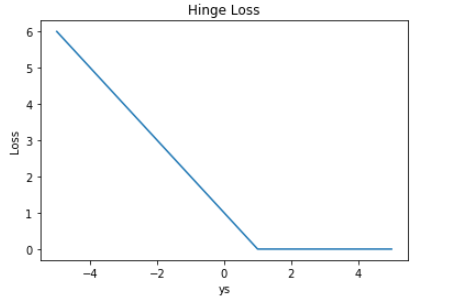Hinge Loss 的形状就像一本要合上的书，故称为合页损失。显然，只有当 ys < 1 时，Loss 才大于零；对于 ys > 1 的情况，Loss 始终为零。Hinge Loss 一般多用于支持向量机（SVM）中，体现了 SVM 距离最大化的思想。而且，当 Loss 大于零时，是线性函数，便于梯度下降算法求导。
Hinge Loss 的另一个优点是使得 ys > 0 的样本损失皆为 0，由此带来了稀疏解，使得 SVM 仅通过少量的支持向量就能确定最终超平面。
Exponential Loss
Exponential Loss，又称指数损失，其表达式如下：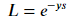可以对上式进行一个直观的理解，类似于上文提到的第二种形式的交叉熵 Loss，去掉 log 和 log 中的常数 1，并不影响 Loss 的单调性。因此，推导得出了 Exponential Loss 的表达式。
Exponential Loss 的曲线如下图所示：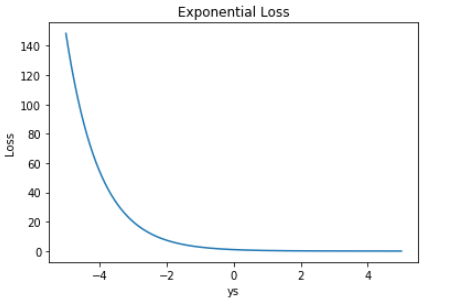Exponential Loss 与交叉熵 Loss 类似，但它是指数下降的，因此梯度较其它 Loss 来说，更大一些。
Modified Huber Loss
Huber Loss，它**结合了均方损失（MSE）和绝对值损失（MAE） ** 的优点，当|y-f(x)|小于一个实现指定的δ时，变为平方损失，大于δ时变为绝对值损失，比前两者更为robust。而Huber Loss 也能应用于分类问题中，称为 Modified Huber Loss，其表达是如下：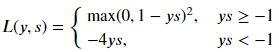Modified Huber Loss 的曲线如下图所示：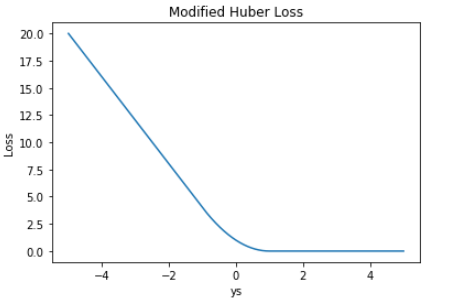从表达式和 Loss 图形上看，Modified Huber Loss 结合了 Hinge Loss 和 交叉熵 Loss 的优点。一方面能在 ys > 1 时产生稀疏解提高训练效率；另一方面对于 ys < −1 样本的惩罚以线性增加，这意味着受异常点的干扰较少。scikit-learn 中的 SGDClassifier 就使用了 Modified Huber Loss。
Softmax Loss
对于多分类问题，也可以使用 Softmax Loss。
机器学习模型的 Softmax 层，正确类别对于的输出是：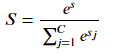其中，C 为类别个数，小写字母 s 是正确类别对应的 Softmax 输入，大写字母 S 是正确类别对应的 Softmax 输出。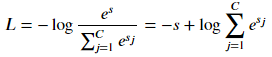Softmax Loss 的曲线如下图所示：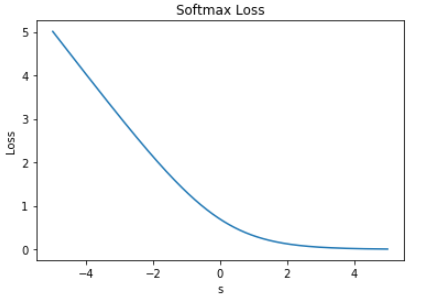上图中，当 s << 0 时，Softmax 近似线性；当 s>>0 时，Softmax 趋向于零。Softmax 同样受异常点的干扰较小，多用于神经网络多分类问题中。
最后，我们将 0-1 Loss、Cross Entropy Loss、Hinge Loss、Exponential Loss、Modified Huber Loss 画在一张图中：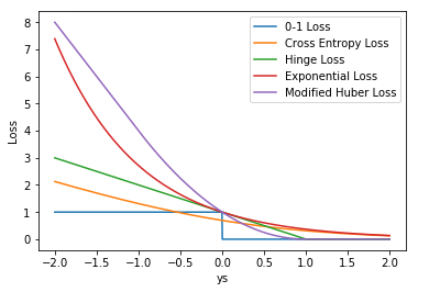从上图可以看出上面介绍的损失函数都可以看作0-1损失的单调连续近似函数，而因为这些损失函数为凸的连续函数，可以用来替代0-1损失进行优化，其相同点在于margin趋向于负无穷时加大“惩罚”；不同点在于，交叉熵损失和合页损失都是线性增长，而指数损失是以指数增长：
上图 ys 的取值范围是 [-2,+2]，若我们把 ys 的坐标范围取得更大一些，上面 5 种 Loss 的差别会更大一些，见下图：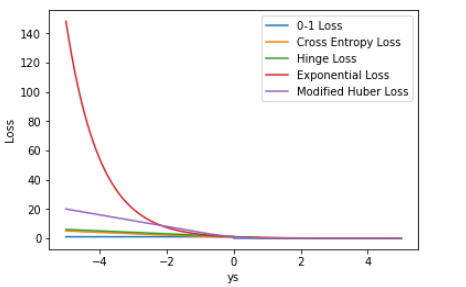显然，这时候 Exponential Loss 会远远大于其它 Loss。从训练的角度来看，模型会更加偏向于惩罚较大的点，赋予其更大的权重。如果样本中存在离群点，Exponential Loss 会给离群点赋予更高的权重，但却可能是以牺牲其他正常数据点的预测效果为代价，可能会降低模型的整体性能，使得模型不够健壮（robust）。
相比 Exponential Loss，其它四个 Loss，包括 Softmax Loss，都对离群点有较好的“容忍性”，受异常点的干扰较小，模型较为健壮。


展开全文• ## 交叉熵损失函数原理详解

万次阅读 多人点赞 2019-08-30 10:28:42
之前在代码中经常看见交叉熵损失函数(CrossEntropy Loss)，只知道它是分类问题中经常使用的一种损失函数，对于其内部的原理总是模模糊糊，而且一般使用交叉熵作为损失函数时，在模型的输出层总会接一个softmax函数，...
交叉熵损失函数原理详解
之前在代码中经常看见交叉熵损失函数(CrossEntropy Loss)，只知道它是分类问题中经常使用的一种损失函数，对于其内部的原理总是模模糊糊，而且一般使用交叉熵作为损失函数时，在模型的输出层总会接一个softmax函数，至于为什么要怎么做也是不懂，所以专门花了一些时间打算从原理入手，搞懂它，故在此写一篇博客进行总结，以便以后翻阅。

交叉熵简介
交叉熵是信息论中的一个重要概念，主要用于度量两个概率分布间的差异性，要理解交叉熵，需要先了解下面几个概念。

信息量
信息奠基人香农（Shannon）认为“信息是用来消除随机不确定性的东西”，也就是说衡量信息量的大小就是看这个信息消除不确定性的程度。
“太阳从东边升起”，这条信息并没有减少不确定性，因为太阳肯定是从东边升起的，这是一句废话，信息量为0。
”2018年中国队成功进入世界杯“，从直觉上来看，这句话具有很大的信息量。因为中国队进入世界杯的不确定性因素很大，而这句话消除了进入世界杯的不确定性，所以按照定义，这句话的信息量很大。
根据上述可总结如下：信息量的大小与信息发生的概率成反比。概率越大，信息量越小。概率越小，信息量越大。
设某一事件发生的概率为P(x)，其信息量表示为：
$I\left ( x \right ) = -\log\left ( P\left ( x \right ) \right )$
其中$I\left ( x \right )$表示信息量，这里$\log$表示以e为底的自然对数。

信息熵
信息熵也被称为熵，用来表示所有信息量的期望。
期望是试验中每次可能结果的概率乘以其结果的总和。
所以信息量的熵可表示为：（这里的$X$是一个离散型随机变量）
$H\left ( \mathbf{X} \right ) = -\sum \limits_{i=1}^n P(x_{i}) \log \left ( P \left ( x_{i} \right ))) \qquad ( \mathbf{X}= x_{1},x_{2},x_{3}...,x_{n} \right)$
使用明天的天气概率来计算其信息熵：

序号
事件
概率P
信息量

1
明天是晴天
0.5
$-\log \left ( 0.5 \right )$

2
明天出雨天
0.2
$-\log \left ( 0.2 \right )$

3
多云
0.3
$-\log \left ( 0.3 \right )$

$H\left ( \mathbf{X} \right ) = -\left ( 0.5 * \log \left ( 0.5 \right ) + 0.2 * \log \left ( 0.2 \right ) + 0.3 * \log \left ( 0.3 \right ) \right)$
对于0-1分布的问题，由于其结果只用两种情况，是或不是，设某一件事情发生的概率为$P\left ( x \right )$，则另一件事情发生的概率为$1-P\left ( x \right )$，所以对于0-1分布的问题，计算熵的公式可以简化如下：
$H\left ( \mathbf{X} \right ) = -\sum \limits_{n=1}^n P(x_{i}\log \left ( P \left ( x_{i} \right )) \right) \\ = -\left [ P\left ( x \right) \log \left ( P\left ( x \right ) \right ) + \left ( 1 - P\left ( x \right ) \right) \log \left ( 1-P\left ( x \right ) \right ) \right] \\ = -P\left ( x \right) \log \left ( P\left ( x \right ) \right ) - \left ( 1 - P\left ( x \right ) \right) \log \left ( 1-P\left ( x \right ) \right)$

相对熵（KL散度）
如果对于同一个随机变量$X$有两个单独的概率分布$P\left(x\right)$和$Q\left(x\right)$，则我们可以使用KL散度来衡量这两个概率分布之间的差异。
下面直接列出公式，再举例子加以说明。
$D_{KL}\left ( p || q \right) = \sum \limits_{i=1}^n p\left ( x_{i}\right ) \log \left ( \frac{p\left ( x_{i} \right )}{q\left ( x_{i} \right )} \right )$
在机器学习中，常常使用$P\left(x\right)$来表示样本的真实分布，$Q \left(x\right)$来表示模型所预测的分布，比如在一个三分类任务中（例如，猫狗马分类器），$x_{1}, x_{2}, x_{3}$分别代表猫，狗，马，例如一张猫的图片真实分布$P\left(X\right) = [1, 0, 0]$, 预测分布$Q\left(X\right) = [0.7, 0.2, 0.1]$，计算KL散度：
$D_{KL}\left ( p || q \right) = \sum \limits_{i=1}^n p\left ( x_{i}\right ) \log \left ( \frac{p\left ( x_{i} \right )}{q\left ( x_{i} \right )} \right ) \\ = p\left ( x_{1}\right ) \log \left ( \frac{p\left ( x_{1} \right )}{q\left ( x_{1} \right )} \right ) + p\left ( x_{2}\right ) \log \left ( \frac{p\left ( x_{2} \right )}{q\left ( x_{2} \right )} \right ) + p\left ( x_{3}\right ) \log \left ( \frac{p\left ( x_{3} \right )}{q\left ( x_{3} \right )} \right ) \\ = 1 * \log \left ( \frac{1}{0.7} \right ) = 0.36$
KL散度越小，表示$P\left(x\right)$与$Q\left(x\right)$的分布更加接近，可以通过反复训练$Q\left(x \right)$来使$Q\left(x \right)$的分布逼近$P\left(x \right)$。

交叉熵
首先将KL散度公式拆开：
$D_{KL}\left ( p || q \right) = \sum \limits_{i=1}^n p\left ( x_{i}\right ) \log \left ( \frac{p\left ( x_{i} \right )}{q\left ( x_{i} \right )} \right ) \\ = \sum \limits_{i=1}^n p \left (x_{i}\right) log \left(p \left (x_{i}\right)\right) - \sum \limits_{i=1}^n p \left (x_{i}\right) log \left(q \left (x_{i}\right)\right) \\ = -H \left (p \left(x \right) \right) + \left [-\sum \limits_{i=1}^n p \left (x_{i}\right) log \left(q \left (x_{i}\right)\right) \right]$
前者$H \left (p \left (x \right)\right)$表示信息熵，后者即为交叉熵，KL散度 = 交叉熵 - 信息熵
交叉熵公式表示为：
$H \left (p, q\right) = -\sum \limits_{i=1}^n p \left (x_{i}\right) log \left(q \left (x_{i}\right)\right)$
在机器学习训练网络时，输入数据与标签常常已经确定，那么真实概率分布$P\left(x \right)$也就确定下来了，所以信息熵在这里就是一个常量。由于KL散度的值表示真实概率分布$P\left(x\right)$与预测概率分布$Q \left(x\right)$之间的差异，值越小表示预测的结果越好，所以需要最小化KL散度，而交叉熵等于KL散度加上一个常量（信息熵），且公式相比KL散度更加容易计算，所以在机器学习中常常使用交叉熵损失函数来计算loss就行了。

交叉熵在单分类问题中的应用
在线性回归问题中，常常使用MSE(Mean Squared Error)作为loss函数，而在分类问题中常常使用交叉熵作为loss函数。
下面通过一个例子来说明如何计算交叉熵损失值。
假设我们输入一张狗的图片，标签与预测值如下：

*
猫
狗
马

Label
0
1
0

Pred
0.2
0.7
0.1

那么loss
$loss = -\left ( 0 * \log \left ( 0.2 \right ) + 1 * \log \left ( 0.7 \right ) + 0 * \log \left ( 0.1 \right )\right) = 0.36$
一个batch的loss为
$loss = -\frac{1}{m}\sum \limits_{i=1}^m \sum \limits_{j=1}^n p \left (x_{ij}\right) log \left(q \left (x_{ij}\right)\right)$
其中m表示样本个数。

总结：

交叉熵能够衡量同一个随机变量中的两个不同概率分布的差异程度，在机器学习中就表示为真实概率分布与预测概率分布之间的差异。交叉熵的值越小，模型预测效果就越好。

交叉熵在分类问题中常常与softmax是标配，softmax将输出的结果进行处理，使其多个分类的预测值和为1，再通过交叉熵来计算损失。

参考：
https://blog.csdn.net/tsyccnh/article/details/79163834

THE END展开全文机器学习
• 前言：pytorch中有几个非常容易搞混淆的函数，它们是softmax和log_sof...前言：pytorch中有几个非常容易搞混淆的函数，它们是softmax和log_softmax，CrossEntropyLoss()和NLLLoss()，为了更加彻底的弄清楚，本文将分为两篇文章来说明，第一篇说明原理，第二篇说明用法。
一、二分类损失函数
1.1 从一个简单的实例说起
对于一个二分类问题，比如我们有一个样本，有两个不同的模型对他进行分类，那么它们的输出都应该是一个二维向量,比如：
模型一的输出为：pred_y1=[0.8,0.2]
模型二的输出为：pred_y2=[0.6,0.4]
需要注意的是，这里的数值已经经过了sigmoid激活函数（为什么要这么说，这对于后面理解pytorch的几个函数是有帮助的），所以0.8+0.2=1，
比如样本的真实标签是
true_y=[1,0]
现在我们来求这两个模型对于这一个类别的分类损失，怎么求？先给出二分类损失函数，表达式如下：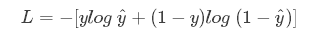这里的y表示的是真实地标签，y上面有一个符号的那个y表示模型的输出标签，我们带入进去计算得到：
cost1 = -[1*log0.8+(1-1)*log0.2] = 0.22314
cost2 = -[1*log0.6+(1-1)*log0.4] = 0.51083
我们可以看出，第一个模型的损失更小，自然我们觉得第一个模型更好，而且直观来看，第一个模型觉得正确的概率是0.8，自然比0.6要好。

二、多分类损失函数
2.1 关键概念——什么是交叉熵
对于连续性的概率分布而言，首先来看信息论中交叉熵的定义：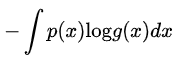交叉熵是用来描述两个分布的距离的，神经网络训练的目的就是使 g(x) 逼近 p(x)。
对于离散型的概率分布而言，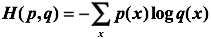注意：交叉熵是一个信息论中的概念，它原来是用来估算平均编码长度的。给定两个概率分布p和q，通过q来表示p的交叉熵为上式，交叉熵刻画的是两个概率分布之间的距离，或可以说它刻画的是通过概率分布q来表达概率分布p的困难程度，p代表正确答案，q代表的是预测值，交叉熵越小，两个概率的分布约接近。

2.2 多分类损失的简单例子
举个例子，假设有一个3分类问题，某个样例的正确答案是（1，0，0），这个模型经过softmax回归之后的预测答案是（0.5，0.4，0.1），
这里的数值已经经过了softmax激活函数（为什么要这么说，这对于后面理解pytorch的几个函数是有帮助的）
那么预测和正确答案之间的交叉熵为：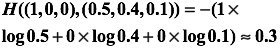如果另一个模型的预测是（0.8，0.1，0.1），那么这个预测值和真实值之间的交叉熵是：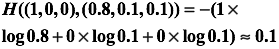显然我们看到第一个模型的损失为0.3，二第二个模型的损失为0.1，第二个模型的损失更小，第二个预测要优于第一个。这里的（1，0，0）就是正确答案p，（0.5，0.4，0.1）和（0.8，0.1，0.1）就是预测值q，显然用（0.8，0.1，0.1）表达（1，0，0）的损失更小一些，准确度更高一些。
下面给一个多分类交叉熵损失函数的一般表达式：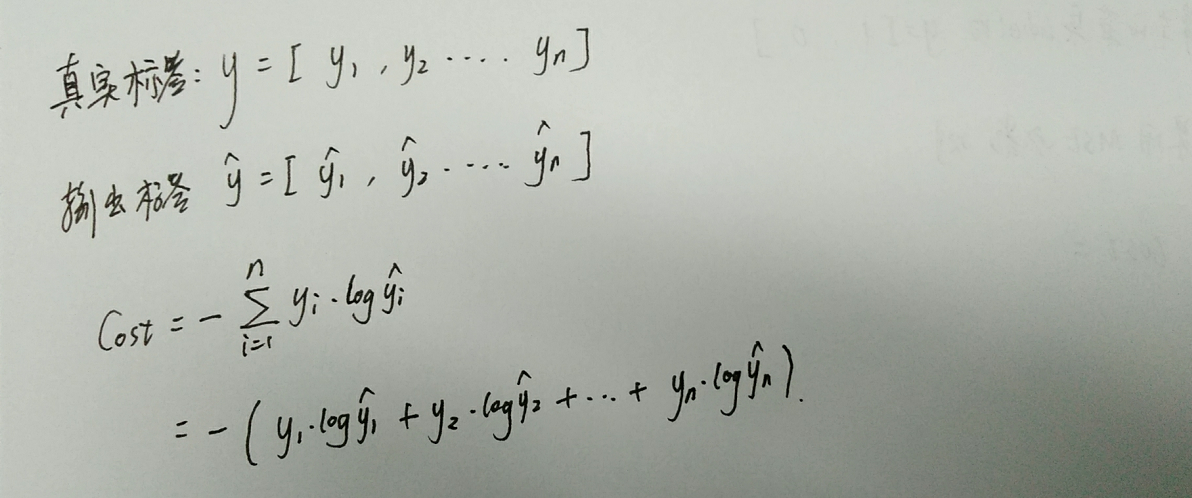总结：不管是二分类，还是多分类问题，其实在计算损失函数的过程都经历了三个步骤：
（1）激活函数。通过激活函数sigmoid或者是softmax将输出值缩放到[0,1]之间，
（2）求对数。计算缩放之后的向量的对数值，即所谓的logy的值，求对数之后的值在[-infinite,0]之间
（3）累加求和。根据损失函数的定义，将标签和输出值逐元素相乘再求和，最后再添加一个负号求相反数，得到一个正数损失。
不管什么样的实现方式，都会经历这三个步骤，不同的是，可能有的函数会将其中的一个或者是几个步骤封装在一起。

三、二分类损失函数的实现
pytorch中二分类损失函数有两种，它们分别是：
torch.nn.BCELoss()  和   torch.nn.BCEWithLogitsLoss()
BCE这三个字母其实就是binary cross entropy的缩写
他们的区别是：

（1）BCELoss：需要先将最后一层经过sigmoid进行缩放然后再通过该函数
（2）BCEWithLogitsLoss：BCEWithLogitsLoss就是把Sigmoid-BCELoss合成一步，不再需要在最后经过sigmoid进行缩放，直接对最后得到的logits进行处理。
备注：logits，指的是还没有经过sigmoid和softmax缩放的结果哦！

四、softmax、log_softmax、CrossEntropyLoss 、NLLLoss 四个函数的对比
（1）softmax/sigmoid
这个只对应于上面的第一步骤，即相当于是激活函数操作，将输出缩放到[0,1]之间
（2）log_softmax
在softmax的结果上再做多一次log运算，即相当于是一次性完成第一步和第二步。
（3）nll_loss
这个实际上只对应于上面的第三个步骤，
（4）CrossEntropyLoss
CrossEntropyLoss就是把以上Softmax–Log–NLLLoss合并成一步
总结：上面的四种方法只是完成的功能，在具体的写代码的时候，还需要我们可以有两种方式来实现上面的四个功能，即通过函数的形式和通过类的形式，如下：
import torch.nn.functional as F

F.xxx          # 通过函数的形式
torch.nn.xxx   # 通过类的形式

总结：通过上面的分析，我们知道了，求多分类交叉熵损失有三种途径可以实现，分别是：
（1）三步实现：softmax+log+nll_loss
（2）两步实现：log_softmax+nll_loss
（3）一步实现：crossEntropyLoss

数据准备：
import numpy as np
import torch
import torch.nn.functional as F

# 比如这是一个模型的输出，本案例为一个三类别的分类，共有四组样本，如下：
pred_y = np.array([[ 0.30722019 ,-0.8358033 ,-1.24752918],
[ 0.72186664 , 0.58657704 ,-0.25026393],
[ 0.16449865 ,-0.44255082 , 0.68046693],
[-0.52082402 , 1.71407838 ,-1.36618063]])
pred_y=torch.from_numpy(pred_y)

# 真实的标签如下所示
true_y = np.array([[ 1 , 0 , 0],
[ 0 , 1 , 0],
[ 0 , 1 , 0],
[ 0 , 0 , 1]])
true_y=torch.from_numpy(true_y)
target = np.argmax(true_y, axis=1) #（4,） #其实就是获得每一给类别的整数值，这个和tensorflow里面不一样哦 内部会自动转换为one-hot形式
target = torch.LongTensor(target)  #（4,）

print(target)            # tensor([0,1,1,2])
print("-----------------------------------------------------------")

4.1 三步实现：softmax+log+nll_loss
如下：
# 第一步：使用激活函数softmax进行缩放
pred_y = F.softmax(pred_y,dim=1)
print(pred_y)
print('-----------------------------------------------------------')

# 第二步：对每一个缩放之后的值求对数log
pred_y=torch.log(pred_y)
print(pred_y)
print('-----------------------------------------------------------')

# 第三步：求交叉熵损失
loss=F.nll_loss(pred_y,target)
print(loss)  # 最终的损失为：tensor(1.5929, dtype=torch.float64)

4.2 两步实现：log_softmax+nll_loss
如下：
# 第一步：直接使用log_softmax,相当于softmax+log
pred_y=F.log_softmax(pred_y,dim=1)
print(pred_y)
print('-----------------------------------------------------------')

# 第二步：求交叉熵损失
loss=F.nll_loss(pred_y,target)
print(loss) # tensor(1.5929, dtype=torch.float64)

注意：使用log_softmax后也可以再使用crossEntropy，效果是等价的，虽然crossEntropy内置了log_softmax。因为重复使用多次log_softmax，结果是不变的，即和使用一次是一样的。不过一般不这么使用。
4.3 一步实现：crossEntropyLoss
# 第一步：求交叉熵损失一步到位
loss=F.cross_entropy(pred_y,target)
print(loss)  tensor(1.5929, dtype=torch.float64)

4.4 总结，在求交叉熵损失的时候，需要注意的是，不管是使用 nll_loss函数，还是直接使用cross_entropy函数，都需要传递一个target参数，这个参数表示的是真实的类别，对应于一个列表的形式而不是一个二维数组，这个和tensorflow是不一样的哦！（Pytorch分类损失函数内部会自动把列表形式(一维数组形式)的整数索引转换为one-hot表示）


展开全文深度学习
• 叙：损失函数的一般表示为 L(y,f(x))，为了便于不同损失函数的比较，常将其表示为单变量的函数，在回归问题中这个变量为y−f(x)，在分类问题中则为yf(x)。 1.回归问题损失函数 回归问题中y和f(x)皆为实数∈R，因此...
参考文章：
https://www.cnblogs.com/massquantity/p/8964029.html
叙：损失函数的一般表示为 L(y,f(x))，为了便于不同损失函数的比较，常将其表示为单变量的函数，在回归问题中这个变量为y−f(x)，在分类问题中则为yf(x)。
1.回归问题的损失函数
回归问题中y和f(x)皆为实数∈R，因此用残差 y−f(x)来度量二者的不一致程度。
常见的回归损失函数有：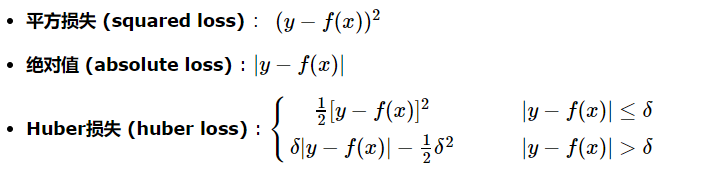Huber损失是对二者的综合，当|y−f(x)|小于一个事先指定的值δ时，变为平方损失，大于δ时，则变成类似于绝对值损失，因此也是比较robust的损失函数
2.分类问题的损失函数
对于二分类问题，y∈{−1,+1}，损失函数常表示为关于yf(x)的单调递减形式。如下图：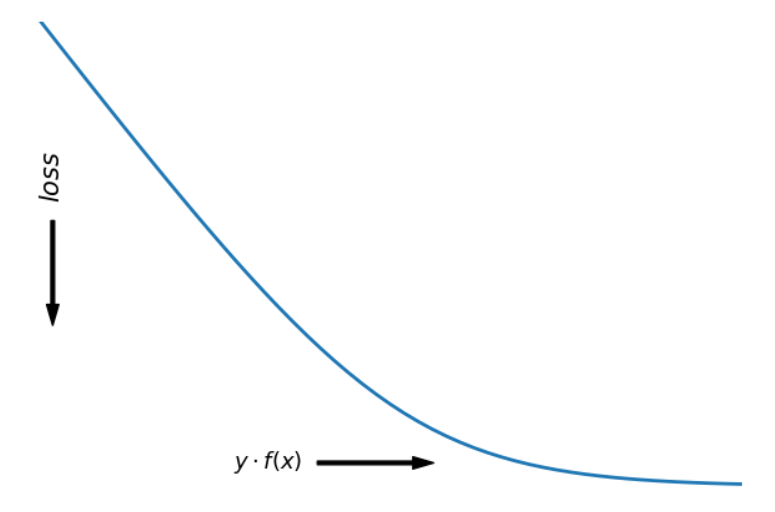yf(x)被称为margin，其作用类似于回归问题中的残差 y−f(x)。可以看到如果yf(x)>0，则样本分类正确，yf(x)<0 则分类错误，而相应的分类决策边界即为f(x)=0。所以最小化损失函数也可以看作是最大化margin的过程，任何合格的分类损失函数都应该对margin<0的样本施以较大的惩罚。
二分类问题常采用sigmoid(f(x))。
常用：
1）0-1损失：
yf(x)|>=0,<0|,0-1损失对每个错分类点都施以相同的惩罚.另外0-1损失不连续、非凸，优化困难，因而常使用其他的代理损失函数进行优化。
2) Logistic loss: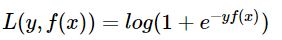logistic Loss为Logistic Regression中使用的损失函数。
令t=(y+1)/2,则t取值|0,1|，则对数似然函数为(下图是式中用t代替y)：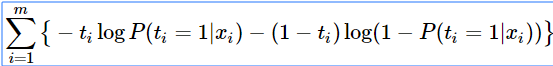上式被称为交叉熵损失 (cross entropy loss)，可以看到在二分类问题中logistic loss和交叉熵损失是等价的，二者区别只是标签y的定义不同。
3）Hinge loss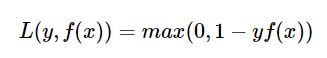hinge loss为svm中使用的损失函数，hinge loss使得yf(x)>1的样本损失皆为0，由此带来了稀疏解，使得svm仅通过少量的支持向量就能确定最终超平面。svm 这个形式的损失函数是自带参数 w 的L2 正则的，而相比之下Logistic Regression的损失函数则没有显式的正则化项，需要另外添加。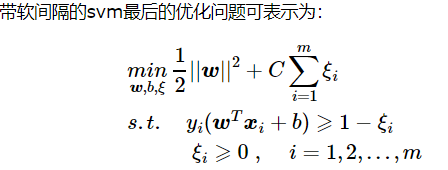4）指数损失(Exponential loss)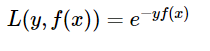5）modified Huber loss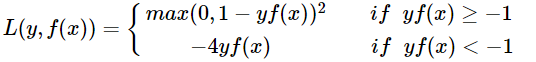modified huber loss结合了hinge loss和logistic loss的优点，既能在yf(x)>1时产生稀疏解提高训练效率，又能进行概率估计。另外其对于(yf(x)<−1) 样本的惩罚以线性增加，这意味着受异常点的干扰较少，比较robust。scikit-learn中的SGDClassifier同样实现了modified huber loss。
分割线：
为什么在神经网络中很多运用交叉熵作为损失函数：
参考：https://zhuanlan.zhihu.com/p/70804197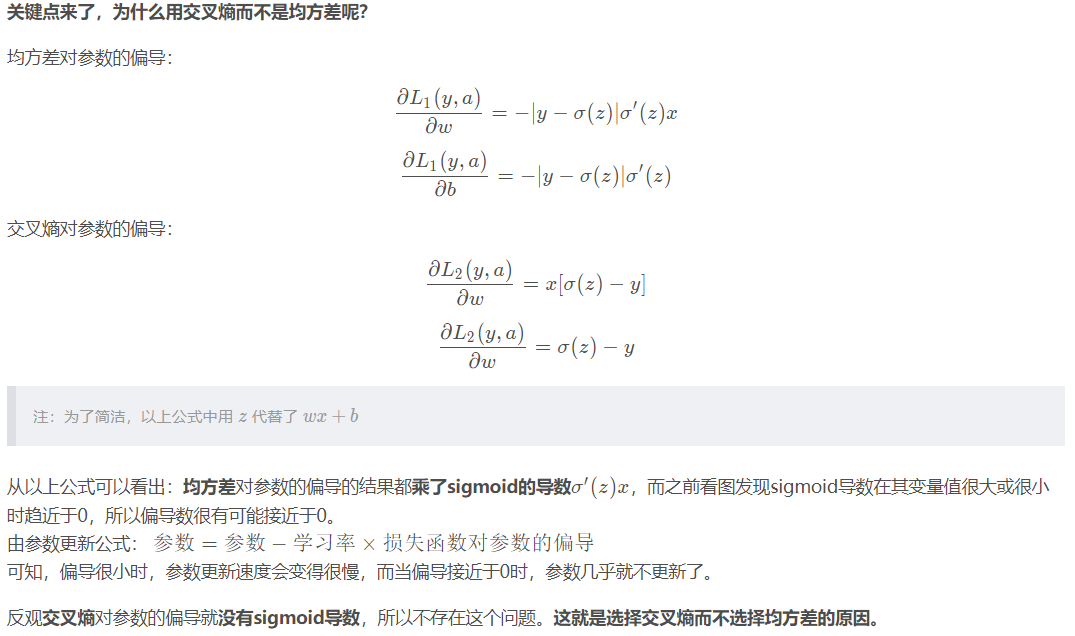展开全文• sigmoid二分类函数：将得分结果转换为概率进行分类。输入x属于任意实数值，输出...损失函数损失函数代表预测值与理想值之间的差值，这个差值越小 ，代表预测值越接近理想值，间接说明我们的网络模型的权重参数比...
• 使用神经网络处理多分类任务时，一般采用 softmax 作为输出层的激活函数，使用categorical_crossentropy（多类别交叉熵损失函数）作为损失函数，输出层包含k个神经元对应k个类别。 在多标签分类任务中，一般采用 ...
• 要建立一个模型，不可或缺的有：1、数据，2、损失函数，3、模型算法，4、优化算法。 今天我们讨论下损失函数这块。损失函数的设计，与模型最后输出的内容是有一定关联的。 对于大部分的任务（回归 or 分类）,最大...
• 解决多标签多分类损失函数选择及样本不均衡问题的2个帖子 https://cloud.tencent.com/developer/ask/226097 https://discuss.pytorch.org/t/multi-label-multi-class-class-imbalance/37573 主要弄明白 nn....pytorch
• author:DivinerShi线性分类损失函数与最优化假设有3类 cat car frog第一列第二行的5.1表示真实类别为cat，然后分类器判断为car的的分数为5.1。那这里的这个loss怎么去计算呢？这里就要介绍下SVM的损失函数，叫...深度学习 优化
• y表示对应的标签，J就可以理解为用二范数的方式将预测和标签的差距表示出来，模型学习的过程就是优化权重参数，使得J达到近似最小值，理论上这个损失函数是很有效果的，但是在实践中却又些问题，它这个h是激活函数...深度学习 机器学习 神经网络
• 分类问题是机器学习中研究和使用都相当多的问题之一；它一般是个有监督的学习(surprised learning)过程；大致来说，有监督的学习是目前工业界有成效应用最多的了，虽然它(surprised learning)距离真正意义上人工...机器学习 编码
• 机器学习中的所有算法都依赖于最小化或最大化一个函数，我们称之为损失函数（loss function），或“目标函数”、“代价函数”。损失函数是衡量预测模型在预测预期结果...损失函数大致可分为两类：分类损失和回归损失，深度学习
• ## 损失函数

千次阅读 2018-10-23 10:27:22
1. 损失函数的定义 机器学习模型关于单个...损失函数大致可分为两类：分类问题损失函数和回归问题损失函数。在这篇文章中，我将着重介绍回归损失。 本文出现的代码和图表我们都妥妥保存在这儿了： https://n...
• 本博文属于阅读笔记，融合了个人观点。 1. 损失函数 损失函数的一般表示为L(y,f(x))，用以衡量真实值 y和...为了便于不同损失函数的比较，常将其表示为单变量的函数，在回归问题中这个变量为[y-f(x)] ：残差表...
• 分类问题是监督学习中最基本的问题之一，本文旨在从评分值、损失函数的角度，对解决二分类问题的诸多机器学习算法做一个梳理。相关算法的详细介绍可以参考相关教材。 给定一个数据集 ，其中，为d维向量 。...
• PyTorch提供了丰富的损失函数，而多分类任务用到最多的就是nn.CrossEntropyLoss和nn.NLLLoss了，不妨讨论一下。 nn.CrossEntropyLoss CrossEntropy顾名思义就是交叉熵，概念来自香农的信息论，用于度量两个概率...
• 文章目录起因原因最根本的原因交叉熵损失函数原理另一个次要原因用交叉熵损失函数后还会有梯度消失的问题吗？参考来源链接 起因 因为最开始听一个老师讲的时候把这个问题将偏了，或者说没有说明根本原因，所以现在这...
• 分类问题和回归问题是监督学习的两大种类：分类问题的目标变量是离散的；回归问题的目标变量是连续的数值。 神经网络模型的效果及优化的目标是通过损失函数来定义的。...对于回归问题，常用的损失函数是均方...AI
• 分类、多分类与多标签问题及对应损失函数的选择 之前只是了解了一些图像分类的知识，知道它大概的意思，因为笔者的研究最终也可以叫做分类，所以今天有时间来一起整理下图像分类以及对应的损失函数。开始学习~????...
• 这个我看过吴恩达的机器学习视频上说过，一般平方损失函数的公式如下图所示： h表示的是你的预测结果，y表示对应的标签，J就可以理解为用二范数的方式将预测和标签的差距表示出来，模型学习的过程就是优化权重...
• 分类问题损失函数——用????????(????)来判断1.0 margin: ????????(????)1.1 0-1损失 (zero-one loss)1.2 Logistic loss1.3 Hinge loss回归问题损失函数——用????−????(????)来度量 写在前面： 损失函数的...
• 损失函数 损失函数，又叫目标函数，是编译一个神经网络模型必须的两个参数之一。另一个必不可少的参数是优化器。 损失函数是指用于计算标签值和预测值之间差异的函数，在机器学习过程中，有多种损失函数可供选择，...
• 一、Softmax函数的导数 Softmax函数公式： Si 代表的是第i个神经元的输出 其中wij 是第i个神经元的第 ...多分类损失函数公式： 其中yi表示真实的分类结果 利用损失函数求梯度 已知不做推导 求解书过程推导： 如果i自然语言处理 神经网络 深度学习
• ## 损失函数——交叉熵损失函数

千次阅读 多人点赞 2019-05-06 09:38:50
损失函数——交叉熵损失函数 摘录： https://zhuanlan.zhihu.com/p/35709485 http://jackon.me/posts/why-use-cross-entropy-error-for-loss-function/ 1.分类模型 与 Loss 函数的定义 监督学习的 2 大分支： ...softmax
• 二值交叉熵是二分类问题的默认损失函数，用于目标变量满足{0，1}二值分布的二分类模型中。 交叉熵回顾——交叉熵是信息理论学里面，用来衡量两个概率分布之间的不相似度，交叉熵越小，概率分布就更相似。 参照...
• 对于机器学习中常见的损失函数有：平方损失函数与交叉熵损失函数。在本文中将讲述两者含义与响应的运用区别。 2. 平方损失函数 平方损失函数较为容易理解，它直接测量机器学习模型的输出与实际结果之间的距离。...
• pytorch学习笔记——二分类交叉熵损失函数分类交叉熵函数 二分类交叉熵函数 binary_cross_entropy 公式： loss=−y∗log⁡(y^)−(1−y)∗log(1−y^) loss=-y\ast \log \left( \widehat {y}\right) -\left( 1-y\...pytorch 深度学习
• 分类即预测未知数据的类别，分类模型的输出可以是离散的，也可以是连续的，一般是输出每个类别的概率，分类分为二元分类和多元分类，二元分类如逻辑回归（LR）、支持向量机（SVM），多元分类如SoftMax、决策树（ID3...
• 交叉熵计算实例： 假设有一个三分类问题，某个样例的正确答案是(1，0，0)，某个模型经过softmax回归之后的预测答案是(0.5，0.4，0.1)，那么他们的交叉熵为： 如果另一个模型的预测概率分布为(0.8，0.1，0.1)，则这......